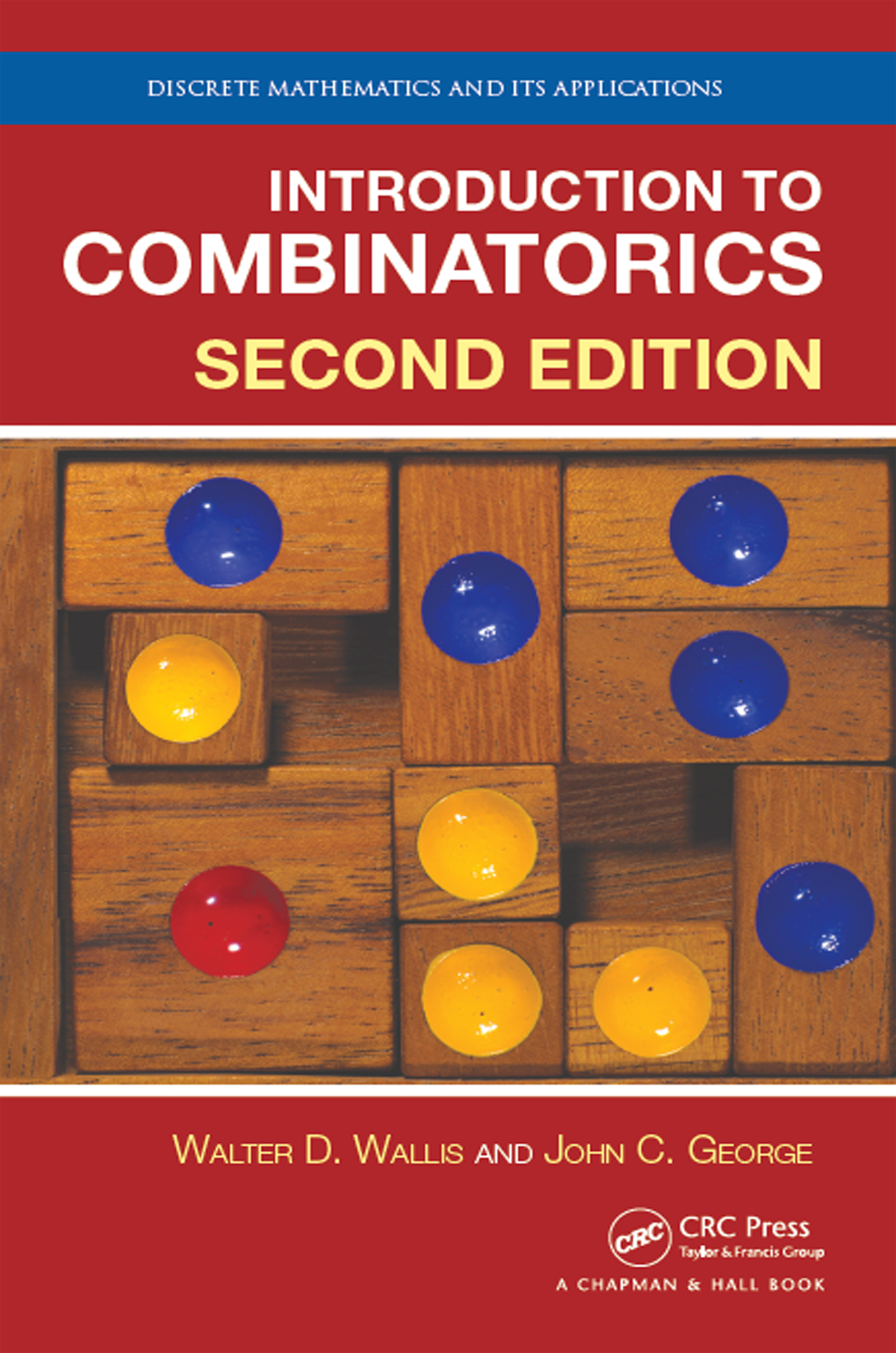# Introduction to Combinatorics

## 2nd Edition

Chapman and Hall/CRC

424 pages | 214 B/W Illus.

##### Purchasing Options:\$ = USD
Hardback: 9781498777605
pub: 2017-01-12
SAVE ~\$29.00
\$145.00
\$116.00
x
eBook (VitalSource) : 9781315366890
pub: 2016-12-12
from \$72.50

FREE Standard Shipping!

### Description

What Is Combinatorics Anyway?

Broadly speaking, combinatorics is the branch of mathematics dealing

with different ways of selecting objects from a set or arranging objects. It

tries to answer two major kinds of questions, namely, counting questions: how many ways can a selection or arrangement be chosen with a particular set of properties; and structural

questions: does there exist a selection or arrangement of objects with a

particular set of properties?

The authors have presented a text for students at all levels of preparation.

For some, this will be the first course where the students see several real proofs.

Others will have a good background in linear algebra, will have completed the calculus

stream, and will have started abstract algebra.

The text starts by briefly discussing several examples of typical combinatorial problems

to give the reader a better idea of what the subject covers. The next

chapters explore enumerative ideas and also probability. It then moves on to

enumerative functions and the relations between them, and generating functions and recurrences.,

Important families of functions, or numbers and then theorems are presented.

Brief introductions to computer algebra and group theory come next. Structures of particular

interest in combinatorics: posets, graphs, codes, Latin squares, and experimental designs follow. The

authors conclude with further discussion of the interaction between linear algebra

and combinatorics.

Features

• Two new chapters on probability and posets.
• Numerous new illustrations, exercises, and problems.
• More examples on current technology use
• A thorough focus on accuracy
• Three appendices: sets, induction and proof techniques, vectors and matrices, and biographies with historical notes,
• Flexible use of MapleTM and MathematicaTM

### Table of Contents

Introduction

Some Combinatorial Examples

Sets, Relations and Proof Techniques

Two Principles of Enumeration

Graphs

Systems of Distinct Representatives

Fundamentals of Enumeration

Permutations and Combinations

Applications of P(n, k) and (n k)

□Permutations and Combinations of Multisets

Applications and Subtle Errors

Algorithms

Probability

Introduction

Some Definitions and Easy Examples

Events and Probabilities

Three Interesting Examples

Probability Models

Bernoulli Trials

The Probabilities in Poker

The Wild Card Poker Paradox

The Pigeonhole Principle and Ramsey’s Theorem

The Pigeonhole Principle

Applications of the Pigeonhole Principle

Ramsey’s Theorem — the Graphical Case

Ramsey Multiplicity

Sum-Free Sets

Bounds on Ramsey Numbers

The General Form of Ramsey’s Theorem

The Principle of Inclusion and Exclusion

Unions of Events

The Principle

Combinations with Limited Repetitions

Derangements

Generating Functions and Recurrence Relations

Generating Functions

Recurrence Relations

From Generating Function to Recurrence

Exponential Generating Functions

Catalan, Bell and Stirling Numbers

Introduction

Catalan Numbers

Stirling Numbers of the Second Kind

Bell Numbers

Stirling Numbers of the First Kind

Computer Algebra and Other Electronic Systems

Symmetries and the P´olya-Redfield Method

Introduction

Basics of Groups

Permutations and Colorings

An Important Counting Theorem

P´olya and Redfield’s Theorem

Partially-Ordered Sets

Introduction

Examples and Definitions

Bounds and lattices

Isomorphism and Cartesian products

Extremal set theory: Sperner’s and Dilworth’s theorems

Introduction to Graph Theory

Degrees

Paths and Cycles in Graphs

Maps and Graph Coloring

Further Graph Theory

Euler Walks and Circuits

Application of Euler Circuits to Mazes

Hamilton Cycles

Trees

Spanning Trees

Coding Theory

Errors; Noise

The Venn Diagram Code

Binary Codes; Weight; Distance

Linear Codes

Hamming Codes

Codes and the Hat Problem

Variable-Length Codes and Data Compression

Latin Squares

Introduction

Orthogonality

Idempotent Latin Squares

Partial Latin Squares and Subsquares

Applications

Balanced Incomplete Block Designs

Design Parameters

Fisher’s Inequality

Symmetric Balanced Incomplete Block Designs

New Designs from Old

Difference Methods

Linear Algebra Methods in Combinatorics

Recurrences Revisited

State Graphs and the Transfer Matrix Method

Kasteleyn’s Permanent Method

Appendix 1: Sets; Proof Techniques7

Appendix 2: Matrices and Vectors

Appendix 3: Some Combinatorial People

### About the Authors

W.D. Wallis is Professor Emeritus of Southern Illiniois University. John C George is Asscoiate Professor at Gordon State College.

### Subject Categories

##### BISAC Subject Codes/Headings:
MAT000000
MATHEMATICS / General
MAT036000
MATHEMATICS / Combinatorics